# Logic Blog

#### Analogue Logic 5 - Division & Multiplication

###### Rate this Entry
Analogue Signal Processing
Go Forth & Multiply

Part 5 - Multiplication, Division & More

Last entry we looked at scaling as our first stab at approaching multiplication in the analogue domain. However, the scaling techniques presented were relatively limited - for upscaling we were limited to fixed integer values, and downscaling was limited to certain signals only. We then took what appeared to be a massive detour into the world of sampling of signals and I left you with the promise that the concepts behind the samplers will allow us to get past the notable limitations of our scaling circuits and also push forwards to Multiplication and Division. Today I make good on that promise... Sort of.

------------------------------------------------------------------------------------------------------

Looking back to the high-speed timer-based sampler discussed previously, I highlighted the issue that because we were sampling with such a small time frame the output value of the sampler was actually one third of the input value. At the time, I glossed over this, labeling it an undesirable behaviour and so fixed it, by adding an upscaling stage that followed the sampler.

However, using the ethos that any predictable and reliable behaviour of the LBP tools can (and should) be leveraged to our advantage, this divide-by-3 system is the obvious starting point from which to enhance our scaling abilities, as it allows us to downscale any arbitrary signal, which was something that we failed to do last time.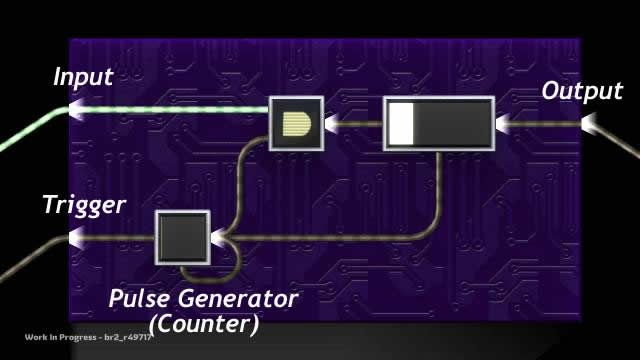fig 1.0.1. High Speed Sampler (from previous blog)

1.1. Sampled Scaling by Rational Numbers
The reason this divide-by-3 came about was because the timer's maximum time was 3-times larger than the sample window created by the pulse generator. As we can keep the same sample window size (of 0.033s) and increase the maximum time on the timer, dividing by larger numbers is possible too.

For example, by setting the timer to 0.2s, we get a ÷6 (because 0.2s / 0.033s = 6) and if we set it to 0.3s we get a ÷9, etc. Indeed, we can divide by any multiple of three, if using the fast sampler. If we were to use the basic (0.1s sample window) sampler, we can actually divide by any integer value - but we shall just ignore that for the moment because it's not half as interesting.

If you combine this with the ability to upscale by any integer (through simple addition of a signal to itself) it's possible to multiply your signal by any rational number.

Just to give a quick example, a scaling factor of 1.5 can be achieved by a mixture of downscaling and upscaling:
1.5 = 3/2
However, we're limited by the requirement of dividing by multiples of 3, so to implement this, we shall use:
1.5 = 9/6

The issue with this is that we have locked ourselves into using a sampling system and the problem with sampling is that you would typically do it at slow intervals. It's generally not great if don't you want a high level of responsiveness. However, technically, the fastest we can push any of our samplers to work is 15Hz, which is actually pretty fast. Not as fast as the 30Hz that everything else runs at, but pretty fast.

To explain where 15Hz comes from: To trigger a sampler, we must see a rising edge on the trigger, which requires that between triggering points, there is a falling edge and then another rising edge (i.e. the state must change from TRUE to FALSE and then back again). 2 changes of state takes 2 system clock cycles, so the period between sample points is 0.066s -> 15Hz. Therefore the maximum frequency is half the frequency of the LBP System Clock (30Hz).Spoiler - geekNotes: On Logic Clocks & Oscillations

If we use the fast sampler, and trigger it at 15Hz, using our astable to trigger the samples, it will reset and return to zero every other clock tick. This would create an output that has valid values only 50% of the time.

Last time we filled in the gaps where our fast sampler was invalid by using a backup sampler. In this case, we could do the same. We'd have to use a fast sampler as the back-up but this would work. With the standard operating frequency of LBP logic being 30Hz, this 15Hz system would be more than adequate for most applications. However, why settle for "adequate"?

1.2. Turbocharged Downscaling
If we go back to the graph above, it should be clear that when running at 15Hz, our fast samplers are valid for 1 clock cycle, then invalid for one clock cycle. The backup sampler would be valid in the clock ticks that the main sampler is invalid for and vice versa. It's not really a main / secondary configuration this time around, they are effectively equal.

So, rather than have the backup sampler sample the main sampler, we could have both of them sample the input directly, and do so on alternate system clock ticks. If we do that and OR the results then the two samplers both only produce valid outputs 50% of the time, but they are offset from one another. When one is invalid, the other becomes valid.

If we have 2 samplers running in antiphase, each valid 50% of the time, and only output whichever is the currently valid value, we will always have valid output. We will also update the output at twice the input clock frequency, giving us 2x15Hz = 30Hz (yay). Plus, watching the circuit below strobe between it's two parallel circuits, while producing a stable value on the output is actually pretty cool!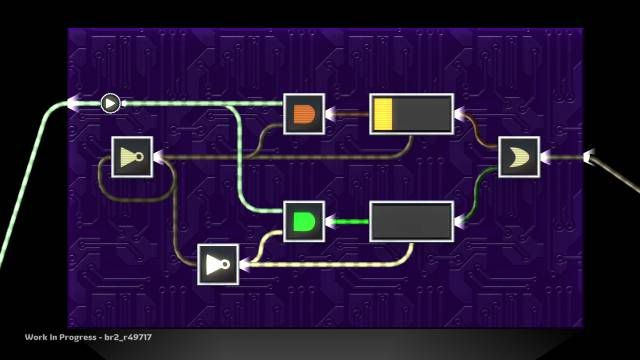fig 1.2.2. Divider Circuit

This is our divider circuit, which is very similar-looking two two of our fast samplers from last time, it's just that the NOT gates are giving us the single-frame pulses that we need for the sample windows. It runs at the system clock rate, the same as the rest of our logic, but it does lag by 0.033s (because our samplers require this to update themselves), but for the sake of squeezing a new function out of the system, that's not too bad and it's very rare that it would cause you an issue. In cases where it does, the issue typically comes from this signal being delayed and other signals not being delayed, giving rise to a race condition. Typically, in the rare occasions where this is the issue it will be acceptable to delay the rest of the signals to bring them in line (more on this later). If the issue with delay is the 0.033s in overall system response, then it's more likely you're just being pernickety, than there is any technical issue.

It's also relatively lightweight once you break it down (it's actually simpler that the 15Hz atomic sampler described in section 1.1). You still still have the issue that you can only divide by integer multiples of 3, but you can of course still multiply this value upwards again to get any value you want. You just have to rejig your fractions so that the denominator is a multiple of three (see example below).

1.3. Variable Coefficient Scaling
So far we've defined scaling as being a multiplication by a fixed value coefficient. However, there is nothing to stop us from creating a system that swaps between two or more scaling coefficients by simply routing the signal through one of several scaling circuits.

Take, for example, a case where you might want to scale by either a half, or a quarter, depending on some condition (for instance a powerup that makes you go faster). We could create complete circuits for both, place them in microchips and then switch between them, but that's a bit complex (especially as you up the number of variants). Instead, for this particular example, we could simply create a system to calculate the divide by four and then conditionally double it, using the techniques we have developed.

To create a divide by 4, we have to use the divider circuit above - the one that only divides by multiples of 3. The best route to take then, is to divide by 12 and then multiply by three, because 1/4 = 3/12 :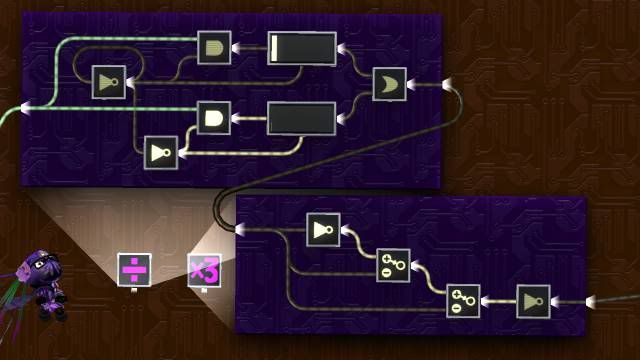fig 1.3.1. Divide by 4 Circuit

Note that the timers are both set to 0.4s. After we have have obtained the divide by four, we can create a conditional doubling circuit by creating a 2 input adder and passing the signal into input 1 and then through input 2 via a relay, as shown (note that the microchip contains the divide by 4 circuit from above, compressed down for clarity).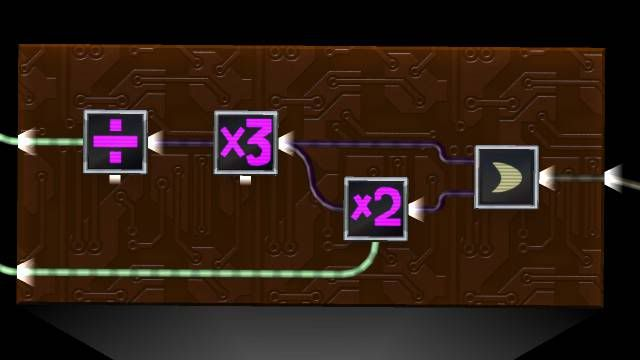fig 1.3.2. Conditional Upscaling Circuit

If the second input is FALSE, then the relay is off and our adder on the right hand side computes:
o = s2 + s3
o = i/4 + 0
o = i/4
However, if we turn on the relay and pass the value through:
o = s2 + s3
o = i/4 + i/4
o = i/2

So if we can conditionally modify the scaling factor by inputting a digital signal to our circuit, and it should be clear that as well as modifying inputs to adders, we can also modify the inputs to min / max circuits and even add in extra dividing stages, for more complex ranges of scaling factors (the above example is quite simple in the fact that the two coefficients are multiples of each other).

------------------------------------------------------------------------------------------------------

2.0. Analogue Multiplication

While we're looking at scaling signals, it seems sensible to attempt the multiplication of 2 analogue signals together. Largely because it's come up in conversation (a while back now, but still), alongside phrases like "not possible", which typically works quite well as a motivator (see: "It's not possible to pass data between levels").

Note: For this, I'm taking the definition of 2-input analogue multiplication within LBP to be based upon percentage values, so 50x50 would give an answer of 25%, not 2,500. This is simply because the limits of analogue signals would make multiplication worthless if the valid answer range was 0-10,000; 99% of the valid values would be out of range of what we can represent on the wire.

2.1. The Supposed Impossibility of Analogue Multiplication
It's been pointed out a couple of times over the beta that multiplication of 2 analogue signals cannot be achieved using the electronic components available to us in LBP2. This is based upon the idea that multiplication is an arithmetic function on analogue values and as it can't be produced using the base analogue functions available, it's not possible. Which all sounds pretty reasonable, in a Mathsy kind of way.

However, it's also come up as an example of a function that can be reproduced using LBP1-esque physical logic, using geometric methods. Theoretically, the geometric method is capable of accurately calculating the product of two values, using triangles. However, the whole concept relies on us being able to convert an analogue signal into distance and back again. Which takes some time to do correctly and is probably never going to be completely accurate in terms of positioning (both factors that affect the validity of the output signal). Therefore, the LBP1 implementation is merely an approximation of multiplication, which is exactly the kind of loophole I need to blag my way through this.

In addition, physical logic being as inefficient as it is, in comparison with LBP2 logic, we've actually got a lot of room, thermo-wise, to play with, if the goal is simply to do a better job than what might be achievable with mechanical means. Hell, I'd settle for doing an ever-so-slightly less good job and take the fact that it can be compressed down into a microchip as enough of a bonus. So, having set the bar suitably low for myself... Let's make this happen:

2.2. Approximating Analogue Multiplication
From previous Blog entries, we know that we can encode analogue data as digital data in arbitrary format. Further up this page, we discussed using digital data to modify the scaling coefficient of another analogue system.

It's not much of a stretch from this to devise a method of approximating the multiplication of two analogue signals. All we need to do is encode one signal (call it input number 2, or i2) into some form of digital data, and then use that digital data to select a scaling coefficient for i1, that is approximately i2.

So, there are a lot of ways that we could approach the encoding of analogue to digital data but for the sake of proof-of-concept, I'm going to go for the garden-variety 7-bit integer encoding that discussed when we looked at ADC. This will give our encoded value to the nearest 1 percent, meaning that our margins of error will be ±0.5. This is where we say that we have an approximation of analogue multiplication. The act of encoding an analogue signal to digital is inherently noisy - the key thing is to know how much noise your application can tolerate.

We encode i2 through a distinctly tedious process of placing batteries in a sequencer (lucky I can go all Blue Peter on you and pull out the one I made earlier, eh? If you don't get the Blue Peter reference don't worry, it's not important - it just means you never had a proper childhood). We then take the s1 and divide it by 100 (achieved by division by 300, followed by multiplication by 3).

* Deep Breath *

The upscaling is where it gets confusing (or confusinger maybe). To do this efficiently, we will want to create 7 different scaled versions of the downscaled input:
• S1
• 2 S1
• 4 S1
• 8 S1
• 16 S1
• 32 S1
• 64 S1

Where S1 is ( i1 / 100 ). After we have produced these, we can switch them on and off using our digital encoding and then sum the results. This is almost exactly the same as how we achieved the 7-bit DAC, but rather than have fixed values weighting the digital inputs (using batteries), we now have values that are downscaled versions i1.

However, rather than producing each one of these weightings individually, we can can just use the fact that each value above is double the previous, so we merely need a cascade of doubling circuits. For this reason, we must always generate each weighting, so that it can be used in the cascade. The weighted value is then conditionally passed into an adder tree, by means of a relay. We can use AND gates relays here, as our "digital" inputs are going to be from batteries inside a sequencer (all set to the default 100%). We also won't have to encounter overflow and we can do a bit of optimisation by removing a few NOT gates here and there in cascade of the doubling circuits. Overall, it comes out as: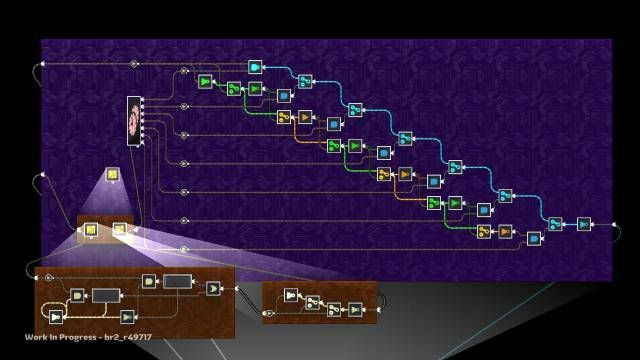fig 1.3.1. Unsigned Multiplier

(apologies for the divider circuit looking slightly different here, that was an editing error on my part... meh)

Note, most of the complexity is contained within the sequencer, as shown below:Spoiler - Oh Mah Gawd :(

2.3. Handling Signed Values
You might think, looking at the complexity of the multiplier above, that a multiplier for signed inputs would be horrendous. Well, it's not. With multiplication we can separate out the calculation of the magnitude and calculation of the sign and then recombine them at the end.

As the sampler used above only works for unsigned values, we will need to obtain the magnitude of that signal - a double NOT gate arrangement (2 NOTs in series) is probably best for this. We don't need to do the same with the digitised input, as the sequencer will carry out the abs() function for us inherently. So the only modification required to the circuit above for evaluating magnitude of signed values is an extra 2 NOT gates.

For the sign of the result, we can obtain signs from both signals using a < 0 comparator circuit, which is very similar to the >0 comparators we used in the ADC blog, and once we have those as a digital values we can carry out an XOR on them. If the XOR returns TRUE, then the answer is negative (as indicated by the truth table below).

Code:
``` Input Sign || Output |
1  |  2  ||  Sign  |
------|-----||--------|
+  |  +  ||    +   |
+  |  -  ||    -   |
-  |  +  ||    -   |
-  |  -  ||    +   |```
Actually, exactly the same result would be given by using >0 comparators... I just wasn't really thinking at the time and it was <0 that I madeWe can force then sign of the analogue magnitude by using AND gate relays, to route the signal into one side of a combiner or the other: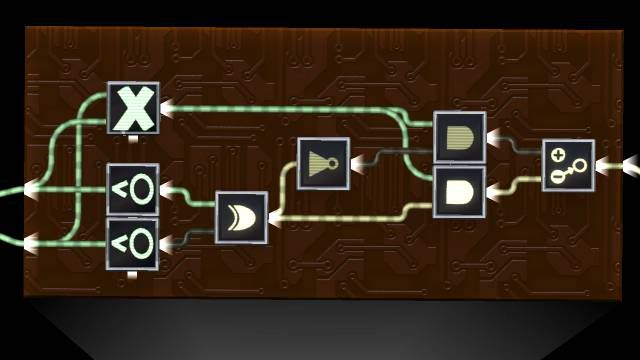fig 1.3.1. Signed Multiplication

Obviously it's still complicated, but considering the cost of the multiplication of magnitudes, the evaluation of sign is pretty much a non-issue.

2.4. Timing considerations
The above is almost a complete analogue multiplication circuit, but at this point it's worth looking into the nature of the timing considerations, notably at the latency introduced by the components, as we are taking a couple of inputs and routing them through a number of reasonably complex circuits to bring them back to a (hopefully) coherent result. We know that at least one of the paths introduces latency, but what about the others?

The divider circuit lags by a single clock tick and then is combined with the digitally encoded signal. However one thing to note about the positional sequencer is that that also lags by a single clock cycle. This is almost certainly a bug in the system, but as we're past release, it's unlikely to get fixed (as it could be level breaking). in this case though, it's kind of useful, as it means that we eliminate race conditions by having both parts of the system lagging by the same amount.

For the case of signed multiplication, the sign values are also generated by positional sequencers (inside those <0 comparators). Therefore these lag as well and conveniently, the entire system inherently syncs up with a single frame of latency. Which is almost convenient enough to think I planned it that way...

2.5. Critique of "Analogue" Multiplication
The above circuit is still pretty complex. It takes up around 1/9th of a small thermo bar, which is a lot for LBP2 logic, especially when you consider that multiplication should really be considered a base function - it's a means to an end, rather than a fully functioning system / subsystem. But then, we're justifying this through comparison to LBP1 logic and for LBP1 logic, 1/9th of a bar isn't unheard of - probably more efficient than a geometric multiplier (which, I'll be honest, I haven't tried to make for comparison). There are a many ways this system could be optimised as well, if you really needed an efficient version. A worst case scenario is that you could simply sacrifice accuracy for efficiency.

On the topic of accuracy, it's worth noting that the ±0.5% accuracy on the digital component is an absolute error - so if your signal is at at 0.5% it will be rounded to 1.0 - which is a 100% proportional error. Probably not an issue, considering that if your signal is at 0.5%, then your output is never going to be greater than 1% anyway, so are you really going to care? If you are, you can probably do a half decent job of encoding signals with better than 1% granularity, using some scaling and / or a really big sequencer. Remembering of course that you'd probably only need to go to that level of detail in the very low range.

2.6. Division
Most mathematical functions come with an inverse: addition and subtraction, min and max, upscaling and downscaling. For 2-input multiplication, the inverse is, of course, division. Dividing one analogue number by another is (sort of) possible using pretty much the same technique as multiplication. It should be clear that division can be characterised by:
o = i1/i2
o = i1 x 1/i2

If we can find a way to create 1/i, then we can indeed use the multiplication technique to provide this functionality. I'd propose that to achieve this, the most sensible approach would be to directly create a digital representation of 1/i . It's an awkward thing to pull off - the shape of the reciprocal curve doesn't really lend itself to being encoded in this way and due to the extremely steep gradient in the first 10%

You're going to need to be careful about exactly how you encode this data. I've not actually done this yet, so I can't really go into details, but it is theoretically possible to achieve a reasonably accurate division function. The key would be to force high resolution along the horizontal axis for low values (think multiple sequencers and up-scale the signal to improve resolution for that region), with lower resolution for the higher values of the input - where the line goes almost horizontal.

------------------------------------------------------------------------------------------------------

3.0. Summary
Quite a lot to take in on this installment, but I think the most important thing is that analogue signal processing has a lot of power behind it. Now that we can simulate the concept of analogue variables, store and recall them at will and carry out a very large number of arithmetic functions on the signals, along with comparisons and conditional switching, we've got most of the fundamental operations that one might find in a programming language. I'm not saying that programming is necessarily a good paradigm to base you LBP2 logic on (I still don't believe that, even as the functional gap betwixt them is reduced), but the point is that once you have a reasonable set of application-agnostic functions, you can achieve pretty much anything you want, in terms of signal manipulation - which is all logic is.

One of the key things to take from this is that ADC and DAC are not just useful as starting points and ending points of analogue circuits. Most of the above are hybrid circuits, with digital and analogue processing interweaved to create new functionality. I still stick by my original concept of keeping a solid line between what is analogue and what is digital - the digital sections are strictly digital and the analogue is strictly analogue and the interactions are explicit conversions (conceptually at least).

One other thing about having a range of fundamental operations, is that you can quickly combine them to create new functions. For a couple of quite advanced examples:

3.1. Arithmetic Mean
The arithmetic mean is defined as the sum of all inputs divided by the number of inputs. We can achieve both of these base functions (summation and division by fixed integer), so therefore the Mean should be a simple thing to process. However, the typically sensible method is to sum first followed by division, which turns out to be pretty much impractical in LBP2. Summation first introduces overflow, which is a pain, so it's best to divide each of the signals first, then sum them together. If a multiply by 3 is required for the downscaling operation, then I suggest doing this after the summation (as you will be doing one x3 rather than an x3 for every input).

3.2. Differentiation
Generally, if you want a real-time analysis of differentiation, then all you need to do is take the current value of the input and subtract it from a value from the past, knowing the time difference between the two. As we can sample values, this is actually very simple to achieve now. However, differentiation works best if the interval between the samples is as short as possible, so with this in mind, it makes sense to make the the time interval a single system clock tick, especially as our divider circuit neatly allows us to lag a signal by exactly this amount. If you divide by three, then multiply by three, you have a divide-by-1 circuit, with an inserted frame of latency. So you merely subtract that from the current value of the signal and you have instantaneous rate of change.

Technically, as our time interval is 0.033s, we should multiply the signal by 30 to achieve a value in Hz (change per second). However, there is no technical reason to use SI units here, if we don't want to: "change per LBP system clock tick" is a perfectly acceptable unit of measurement for rate of change. In addition, a x30 on your signal will overflow for most input values. Of course, if you have a signal that varies quite slowly, you may wish to amplify it for ease of analysis, but in general terms, the primary reason for not amplifying the signal is that you can always guarantee that the unamplified signal will never change by more than 100%, so it will always fit into the range +/- 100% that is output by the combiner.

Signed values are possible by using parrallel x1 circuits (one for the positive, one for the negative. However, this will result in an output range of +/- 200% as the maximum change would be from one extreme to the other in a single clock cycle ( 100 - (-100) = 200 ), so we would need to implement signed subtraction, with overflow handling of some kind.
Categories
Logic Blog

1.Rtm, u CRAAAaazzyyy.
2.Crazy awesome, is what I think you mean3.Originally Posted by rtm223
Crazy awesome, is what I think you meanPerhaps...
4.Another mind-blowingly awesome blog rtm.

pernickety ftw!
5....wow... just .. wow6.Awesome. You officially rock.
7.Yay, a new post! I don't know if you've recreated your analog signal probe from the beta yet, but if not, you might find this useful:

http://www.lbpcentral.com/forums/sho...821#post768821

It's super-accurate, down to the ten-thousandths!

I can tell you how it works if you want.
Updated 02-10-2011 at 12:32 AM by phort
8.Wow. I saw this a few days ago but I've been putting off reading it 'cuz it looked intimidating. I asked you about averaging two signals once and you showed me your circuit: I dismissed it as being too slow to be useful as soon as I saw the timer, not realizing that you were able to achieve a 30Hz sample. Now I feel stupid... er... stupider, though I'm pretty sure the idea I needed averages for would have failed anyway.

Anyway, I can't even count how many times I've wanted an easy/reliable way to downscale a signal and here it is. Yay! I also like that last bit about differentiation: I'm working on a lightsaber and I've had to use a kinda' sloppy technique of emitting keys on holo and tracking their position relative to the saber to get that whooshing noise it makes, but if I run the player's thumbstick through a differentiator, it should be better and more cleanly and I should be able to get better control over the output.

As for the multiplication part, I may take a crack at it later, but for now it's over my head9.@phort: I assume you've got a a system of subtracting values for each digit, then amplifying the signal by 10 to get higher resolution? I started making one of these then found the sequencer latency was annoying. Was gonna update it to remove the latency basically do all subtraction before the evaluation of each digit) but then didn't actually ever get around to it. I don't actually have a working probe in the full game yet@sehven: Yeah, the divider is a weird looking thing and completely unintuitive - it's not something I would have designed from scratch, it only came about through evolution of the samplers. Aya pointed out that the doubel NOT gate configuration was better implemented as a select switch (a point I missed due to evolving design) and phort independently came up with a circuit that is identical in function but with a slightly different configuration, which is worth taking a look at:I prefer my version (obviously), but either works soundly10.Hi analogue geeks,

I've read the blog with much interest: great work!

I'm having a problem with a rotator / joystick issue that I believe is due to a flaw in my analogue logic. If you have any time, could you look at this thread?

Ruisje
11.I have a question. If you for example want to multiply a value by 0.64, does is matter (speedwise) if you map the entire function on a sequencer or if you do 96/150?
12.Should be identical. Divider will add in 1 frame delay, as will the sequencer.

64s an easy number to factor as well, I'd guess you'd be looking at lower thermo, higher accuracy and less ballache with the 96/150.

@Ruisje, if you're still struggling at the weekend I'll try to take a look. Is a bit much for me right now - I'm bloody knackered13.Thanks. I think i'll go with de divider, since thermo isn't really an issue when it comes to most logic.

Lol, i have just been looking for 30 minutes what was wrong with a complex logic circuit that i build, and then i would out i scaled by first multiplying and then dividing, resulting in very weird answers:.
14.Gaaaaaah 8(¯¯¯¯¯¯¯¯¯¯¯¯¯¯¯¯¯¯¯¯¯¯¯¯¯)

This is... Greaaaat! Mmm, i'll look back at my divider (which I didn't build), cause I'm not sure it's accurate. Is yours 100% accurate ? No, cause it uses timers. Right ? I hate the fact that timers aren't precise...
15.this makes no sence O.O